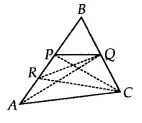Q

# P and Q are respectively the mid-points of sides AB and BC of a triangle ABC and R is the mid-point of AP, show that (ii) ar (RQC) = 3/8 ar (ABC)

Q: 7     P and  Q are respectively the mid-points of sides AB and BC of a triangle ABC and R is the mid-point of AP, show that
(ii)

ViewsIn RBC, RQ is the median
Therefore ar(RQC) = ar(RBQ)
= ar (PRQ) + ar (BPQ)
= 1/8 (ar ABC) + ar(BPQ)  [from eq (vi) & eq (A) in part (i)]
= 1/8 (ar ABC) + 1/2 (ar  PBC)  [ since PQ is the median of BPC]
= 1/8 (ar ABC) + (1/2).(1/2)(ar ABC) [CP is the medain of ABC]
= 3/8 (ar ABC)

Hence proved.

Exams
Articles
Questions